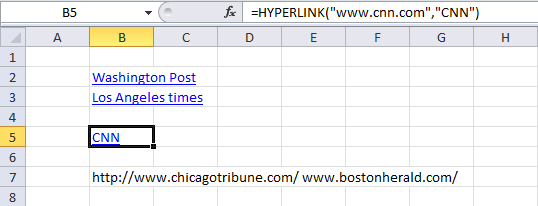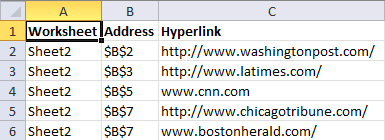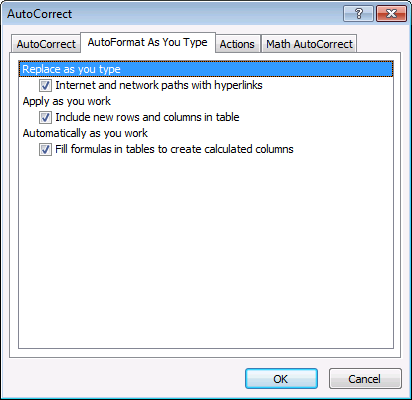Author: Oscar Cronquist Article last updated on September 27, 2019

The macro in this blog post lists all hyperlinks in a worksheet. It also lists all words beginning with "http" or "www". It grabs the hyperlink even if you have a hyperlink function in a cell.

Example, this sheet has two regular hyperlinks (B2:B3) and one hyperlink function (B5) and two web addresses (B7).This is what the macro returns, the worksheet name in column A. Cell address in column B and the hyperlink in column C.### VBA Macro

```Sub ListHyperlinks()
Set Asheet = ActiveSheet
Set Nsheet = Sheets.Add
Nsheet.Range("A1:C1") = Array("Worksheet", "Address", "Hyperlink")
Nsheet.Range("A1:C1").Font.Bold = True

i = 0
For Each cell In Asheet.UsedRange
On Error Resume Next
lnk = cell.Hyperlinks(1).SubAddress
If Err = 0 Then
Nsheet.Range("A2").Offset(i, 0) = Asheet.Name
Nsheet.Range("B2").Offset(i, 0) = cell.Address
Nsheet.Range("C2").Offset(i, 0) = cell.Hyperlinks(1).Address
i = i + 1
Else
If Left(cell.Formula, 11) = "=HYPERLINK(" Then
strArray = Split(cell.Formula, Chr(34))
Nsheet.Range("A2").Offset(i, 0) = Asheet.Name
Nsheet.Range("B2").Offset(i, 0) = cell.Address
Nsheet.Range("C2").Offset(i, 0) = strArray(1)
i = i + 1
Else
strArray = Split(cell)
For Each vl In strArray
If Left(vl, 4) = "http" Or Left(vl, 3) = "www" Then
Nsheet.Range("A2").Offset(i, 0) = Asheet.Name
Nsheet.Range("B2").Offset(i, 0) = cell.Address
Nsheet.Range("C2").Offset(i, 0) = vl
i = i + 1
End If
Next vl
End If
End If
On Error GoTo 0
Next cell

Nsheet.Columns("A:C").AutoFit

End Sub
```

### Where to put the code?

1. Press Alt +F11
2. Insert a module
3. Paste vba code to code window
4. Exit VB Editor Alt + Q

### How to start macro?

1. Press Alt+F8
2. Click "ListHyperlinks"
3. Click "Run" button

### What happens when I run the macro?

1. New sheet is inserted
2. Sheet is populated with hyperlink data

### How do I find all hyperlinks in a workbook?

This macro allows you to find links in all worksheets in active workbook.

```Sub ListHyperlinksInWB()

Set Nsheet = Sheets.Add
Nsheet.Range("A1:C1") = Array("Worksheet", "Address", "Hyperlink")
Nsheet.Range("A1:C1").Font.Bold = True

i = 0
For Each sh In ActiveWorkbook.Worksheets
If sh.Name <> Nsheet.Name Then

For Each cell In sh.UsedRange
On Error Resume Next
lnk = cell.Hyperlinks(1).SubAddress
If Err = 0 Then
Nsheet.Range("A2").Offset(i, 0) = sh.Name
Nsheet.Range("B2").Offset(i, 0) = cell.Address
Nsheet.Range("C2").Offset(i, 0) = cell.Hyperlinks(1).Address
i = i + 1
Else
If Left(cell.Formula, 11) = "=HYPERLINK(" Then
strArray = Split(cell.Formula, Chr(34))
Nsheet.Range("A2").Offset(i, 0) = sh.Name
Nsheet.Range("B2").Offset(i, 0) = cell.Address
Nsheet.Range("C2").Offset(i, 0) = strArray(1)
i = i + 1
Else
strArray = Split(cell)
For Each vl In strArray
If Left(vl, 4) = "http" Or Left(vl, 3) = "www" Then
Nsheet.Range("A2").Offset(i, 0) = sh.Name
Nsheet.Range("B2").Offset(i, 0) = cell.Address
Nsheet.Range("C2").Offset(i, 0) = vl
i = i + 1
End If
Next vl
End If
End If
On Error GoTo 0
Next cell
End If
Next sh

Nsheet.Columns("A:C").AutoFit

End Sub

```

### Download excel *.xlsm file

List hyperlinks.xlsm

Tip! If you want to stop automatic hyperlinking (excel 2010) follow these steps

1. Click "File" on the ribbon
2. Click "Options"
3. Click "Proofing"
4. Click "AutoCorrect Options..."
5. Go to "Autoformat as you type" on the menu
6. Disable "Internet and network paths with hyperlinks"